Courses

# Principal Stress & Strain - MCQ Test 1

## 20 Questions MCQ Test RRB JE for Mechanical Engineering | Principal Stress & Strain - MCQ Test 1

Description
This mock test of Principal Stress & Strain - MCQ Test 1 for Mechanical Engineering helps you for every Mechanical Engineering entrance exam. This contains 20 Multiple Choice Questions for Mechanical Engineering Principal Stress & Strain - MCQ Test 1 (mcq) to study with solutions a complete question bank. The solved questions answers in this Principal Stress & Strain - MCQ Test 1 quiz give you a good mix of easy questions and tough questions. Mechanical Engineering students definitely take this Principal Stress & Strain - MCQ Test 1 exercise for a better result in the exam. You can find other Principal Stress & Strain - MCQ Test 1 extra questions, long questions & short questions for Mechanical Engineering on EduRev as well by searching above.
QUESTION: 1

### A block of steel is loaded by a tangential force on its top surface while the bottom surface is held rigidly. The deformation of the block is due to

Solution:

It is the definition of shear stress. The force is applied tangentially it is not a point load so you cannot compare it with a cantilever with a point load at its free end.

QUESTION: 2

### The figure shows the state of stress at certain point in a stresses body. The magnitudes of normal stresses in x and y direction are 100 MPa and 20 MPa respectively. The radius of Mohr’s stress circle representing this state of stress is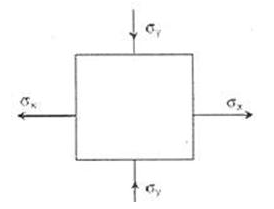Solution:QUESTION: 3

### The state of stress at a point in a loaded member is shown in the figure. The magnitude of maximum shear stress is [1MPa = 10 kg/cm2]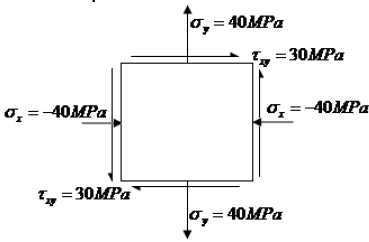Solution: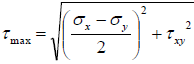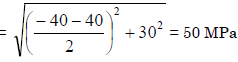QUESTION: 4

In a bi-axial stress problem, the stresses in x and y directions are (σ x = 200 MPa and σy =100 MPa. The maximum principal stress in MPa, is:

Solution: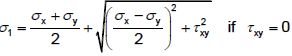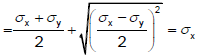QUESTION: 5

The normal stresses at a point are σx = 10 MPa and, σy = 2 MPa; the shear stress at this point is 4MPa. The maximum principal stress at this point is:

Solution: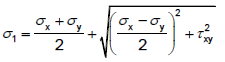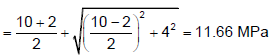QUESTION: 6

The state of stress at a point under plane stress condition is

σxx = 40 MPa , σyy = 100 MPa and τxy = 40 MPa .

The radius of the Mohr‟s circle representing the given state of stress in MPa is

Solution: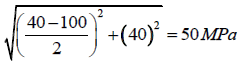QUESTION: 7

When a component is subjected to axial stress the normal stress σn is maximum, if cos θ is _______ . (σnxCos2θ)

1. maximum
2. minimum
3. always one
4. always zero

Solution:
QUESTION: 8

If the two principal strains at a point are 1000 × 10-6 and -600 × 10-6, then the maximum shear strain is:

Solution:

Shear strain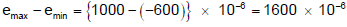QUESTION: 9

In the case of bi-axial state of normal stresses, the normal stress on 45° plane is equal to

Solution: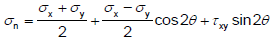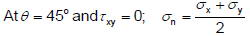QUESTION: 10

In a two-dimensional problem, the state of pure shear at a point is characterized by

Solution:
QUESTION: 11

For the state of stress of pure shear τ the strain energy stored per unit volume in the elastic, homogeneous isotropic material having elastic constants E and ν will be:

Solution: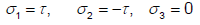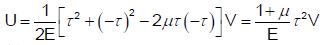QUESTION: 12

Assertion (A): Circular shafts made of brittle material fail along a helicoidally surface inclined at 45° to the axis (artery point) when subjected to twisting moment.

Reason (R): The state of pure shear caused by torsion of the shaft is equivalent to one of tension at 45° to the shaft axis and equal compression in the perpendicular direction.

Solution:

Both A and R are true and R is correct explanation for A.

QUESTION: 13

The state of plane stress in a plate of 100 mm thickness is given as σxx = 100 N/mm2, σyy = 200 N/mm2, Young's modulus = 300 N/mm2, Poisson's ratio = 0.3. The stress developed in the direction of thickness is:

Solution:
QUESTION: 14

Consider the following statements:

State of stress in two dimensions at a point in a loaded component can be completely specified by indicating the normal and shear stresses on

1. A plane containing the point

2. Any two planes passing through the point

3. Two mutually perpendicular planes passing through the point

Of these statements

Solution:
QUESTION: 15

In a strained material one of the principal stresses is twice the other. The maximum shear stress in the same case is tmax . Then, what is the value of the maximum principle stress?

Solution: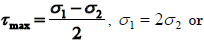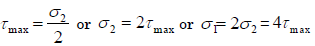QUESTION: 16

The principal stresses σ1, σ2 and σ3 at a point respectively are 80 MPa, 30 MPa and –40 MPa. The maximum shear stress is:

Solution: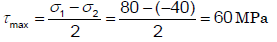QUESTION: 17

Plane stress at a point in a body is defined by principal stresses 3σ and σ. The ratio of the normal stress to the maximum shear stresses on the plane of maximum shear stress is:

Solution: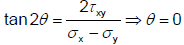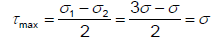Major principal stress on the plane of maximum shear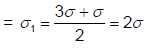QUESTION: 18

For the state of plane stress.
Shown the maximum and minimum principal stresses are: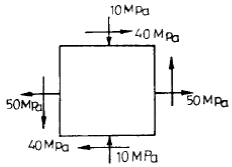Solution: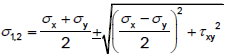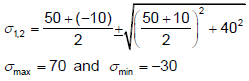QUESTION: 19

Maximum shear stress in a Mohr's Circle

Solution: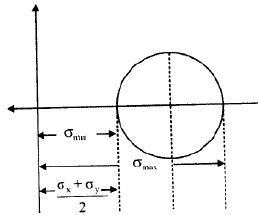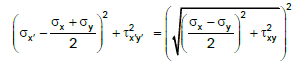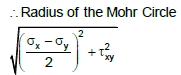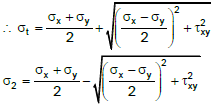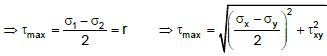QUESTION: 20

Two-dimensional state of stress at a point in a plane stressed element is represented by a Mohr circle of zero radius. Then both principal stresses

Solution: